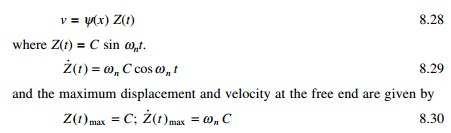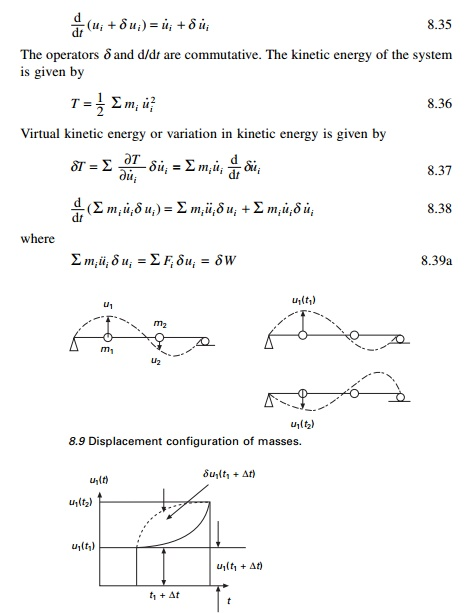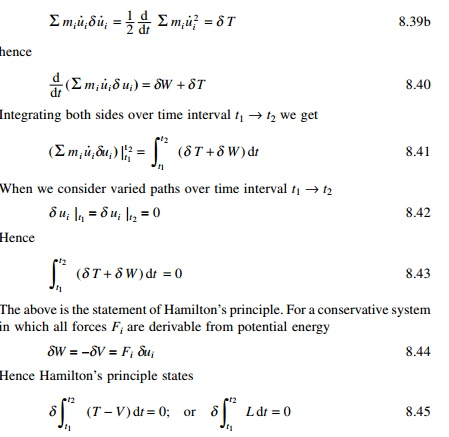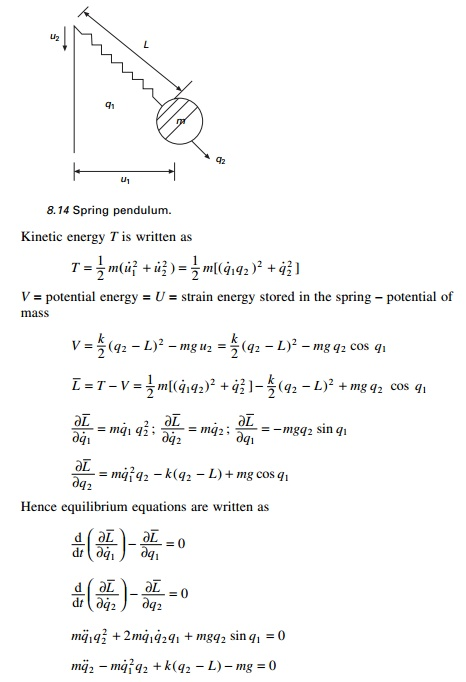Home | | Structural Dynamics and Earthquake Engineering | Generalized coordinates and energy methods

# Generalized coordinates and energy methods

Generalized coordinates and energy methods in relation to structural dynamics during earthquakes: Abstract: A structural system consisting of multiple interconnected mass and flexibilities is modelled as a simple oscillator and the response is studied. The governing equations are derived based on the principle of virtual work. RaleighŌĆÖs method is employed to approximate the fundamental frequency of continuous system. HamiltonŌĆÖs principle is derived and the general dynamic equilibrium equations are obtained using LagrangeŌĆÖs equations. A program in MATHEMATICA is given to obtain the dynamic equilibrium equations once Lagrange equation is given.

Generalized coordinates and energy methods in relation to structural dynamics during earthquakes

Abstract: A structural system consisting of multiple interconnected mass and flexibilities is modelled as a simple oscillator and the response is studied. The governing equations are derived based on the principle of virtual work. RaleighŌĆÖs method is employed to approximate the fundamental frequency of continuous system. HamiltonŌĆÖs principle is derived and the general dynamic equilibrium equations are obtained using LagrangeŌĆÖs equations. A program in MATHEMATICA is given to obtain the dynamic equilibrium equations once Lagrange equation is given.

Key words: virtual work, shape function, generalized mass, Rayleigh method, HamiltonŌĆÖs principle, Lagrange equation.

Introduction

In the earlier chapters, we obtained the response due to dynamic loads of structures modelled as a simple oscillator system. In this chapter, we present the response of a structural system consisting of multiple interconnected rigid bodies or having distributed mass or flexibility. It will be seen that this will also be modelled as a single-degree-of-freedom (SDOF) system. The equations are derived based on principle of virtual work (virtual displacements).

Principle of virtual work

This principle is very useful in establishing dynamic equation of equilibrium for multiple interconnected rigid bodies. This principle was originally developed for equilibrium system but it can also be applied to dynamic system by the simple recourse to DŌĆÖAlembertŌĆÖs principle. The principle of virtual displacement may be stated as follows:

For a system that is in equilibrium, the work done by all the forces during an assumed displacement (virtual displacement) which is compatible with the system constraints is equal to zero.

To illustrate the application of virtual work, let us consider a spring mass├É damper system subjected to external force as shown in Fig. 8.1. Since the inertial forces have been included among the external forces, the system isin equilibrium. If a virtual displacement ╬┤u is assumed to have taken place, the total work done by the forces shown is equal to zero i.e.Since ╬┤u is arbitrary, imaginary not equal to zero, cancelling ╬┤u on both sides we getThus we obtain the differential equation of motion for the damped oscillator.

Generalized SDOF system: rigid bodies

Most frequently the configuration of a dynamic system is specified by coordinates indicating the linear or angular position of elements of the system. Generalized coordinates are independent coordinates with which we define the deformed shape of the structure.

The example of the rigid body shown in Fig. 8.2 consists of a rigid bar with distributed mass m/unit length with rigid mass M at right end. The rod is supported by springs and dampers. Dynamic excitation is provided by a transverse load F(x, t) varying in a triangular fashion as shown in Fig. 8.2. There is also a rotation spring of stiffness k╬Ė at the left end. Our purpose is to obtain the differential equation of motion and to identify the corresponding expressions for parameters of the simple oscillation representing the system.Figures 8.3 and 8.4 represent the true deformed shape and virtual deformed shape diagram. Since it is a rigid bar, the internal work is zero. The external virtual work is calculated as shown in Table 8.1.

Summing up the fourth column of Table 8.1 we get (assuming k╬Ė is zero)Systems having distributed stiffness and distributed mass

For systems idealized with distributed mass and distributed stiffness characteristics, flexural deformations occur that allow the structure to exhibit an infinite degrees of freedom. However, a generalized SDOF analysis can be made if it is assumed that only a single deflection pattern could occur. Hence the motion of the body can be described by a single variable, or generalized coordinate and only one degree of freedom exists. Systems idealized in the manner are referred to as generalized SDOF systems.

Consider a cantilever beam shown in Fig. 8.5. It is assumed that it has distributed mass and distributed stiffness properties. The vertical displacement v at any location on the beam is a function of both position and time, i.e. v(x, t) which can be written as

v(x, t) = Z(t)Žł (x)   ŌĆ”ŌĆ”ŌĆ”. ŌĆ”ŌĆ”ŌĆ” 8.9

where Z(t) is the generalized coordinates representing deflection at the freeend at any time ŌĆśtŌĆÖ and Žł(x) is the shape function. The kinetic energy T of the beam shown in Fig. 8.5 vibrating in the pattern indicated by Eq. 8.9 isThe flexural strain energy U of a prismatic beam may be determined as the work done by the bending moment M(x) undergoing angular displacement d╬Ė. This angular displacement is obtained asFinally, equating the potential energy Eq. 8.16 for the continuous system to the potential energy of the equivalent system, we getThe generalized force F*(t) may be found from the virtual displacements ╬┤Z(t) of the generalized coordinate Z(t) upon equating the work performed by the external forces in the structure to the wok don by the generalized force in the equivalent SDOF system.To calculate the potential energy of the axial force N, which is unchanged during vibration of the beam and consequently is a conservative force, it is necessary to evaluate the horizontal component of the motion ╬┤u(t) at the free end of the beam.Example 8.1

Determine the dynamic equation of motion, natural frequency and buckling load of the water tower shown in Fig. 8.6 with distributed mass m, stiffness EI, concentrated mass at the top = mL. The tower is subjected to earthquake ground motion of u╦Ö╦Ög and an axial compression load at the top. Neglect damping in the system. Assume that during the motion, the shape of the tower is given by Žł(x) = x2/L2.8.5          Rayleigh method

The method is based on the principle of stationary potential energy. Lord Rayleigh in his famous work ŌĆśTheory of soundŌĆÖ published this work in 1877 states that:

ŌĆśIn a natural mode of vibration of a conservative system the frequency of vibration is stationary.ŌĆÖ

The Rayleigh method is the basis for the majority of approximate techniques used in vibration analysis. It may also be employed to approximate the fundamental frequency of continuous systems.

From the previous section (assuming concentrated mass = 0), the displacement at any section X is written asC is the amplitude of vibration at the generalized coordinate location. Now, maximum potential energy is given bywhere ŽēR is known as the Rayleigh frequency.

Obviously, the more closely the assumed shape function Žł(x) approximates the exact vibration mode, the more accurate will be the estimate of the fundamental frequency. As a minimum requirement, the assumed shape function should satisfy the prescribed geometry boundary condition for the problem and also be differentiable at least to the order appearing in the strain energy expression.

Example 8.2

A uniform cantilever beam shown in Fig. 8.5 of length L, and having a flexural rigidity EI, cross-sectional area A and mass density Žü has a concentrated mass M at the free end that is pinned to a linear spring of stiffness k. Determine Žēn by the Rayleigh method. Assume shape function as Žł ( x ) =  1 Ōł' cos  ŽĆ x /2 L where x is measured from the left end.which results in relative error of 4.18%. If Žł is assumed as Žł = x2/L2 would result in relative error of of 27.2%. This indicates that the assumed shape function does not provide an accurate representation of the actual vibration mode.

Improved Rayleigh method

The concept of applying inertial forces as static loads in determining the deformed shape for RayleighŌĆÖs method may be used in developing an improved scheme for the method. In the application of the improved RayleighŌĆÖs method, one would start with assumed deformation curve followed by the calculation of the mass values for kinetic energy and for potential energy of the system. An approximate value of natural frequency is calculated by equating maximum kinetic energy with maximum potential energy. Thus an improved value of natural frequency may be obtained by loading the structure with inertial loads associated with assumed deflection. This load results in a new deformed shape which is used for calculating maximum potential energy.

Example 8.3

Determine using improved RayleighŌĆÖs method the natural frequency of the cantilever beam subjected to concentrated masses (see Fig. 8.7).HamiltonŌĆÖs principle

The Irish mathematician and physicist Sir William Hamilton (1805├É1865) formulated his principle in dynamics in which the governing equation depends explicitly on the energy of the system.

Consider an ideal system of interconnected rigid masses whose displacements are uj j = 1, 2 ├ē n within the interval of time t = t1 to t = t2 as shown in Fig. 8.9. We can plot the displacement ui as a function of time as shown in Fig. 8.10.

The velocity is obtained by taking time derivative asL is termed as Lagrangian or the kinetic potential when strain energy U alone is potential.

HamiltonŌĆÖs principle states that of all the possible paths of motion of a system during the interval of time t1 to t2 the actual path will be the one forLagrangeŌĆÖs equations

The French mathematician Lagrange (1736├É1813) discovered a relationship for providing a method of great power and versatility for the formulation of dynamic equations of equilibrium. Consider a system of connected rigid bodies.Example 8.4

Obtain the dynamic equation of equilibrium using Lagrange equations. m = mass/unit length of the cylinder, L = length of the cylinder, M = mass of the rectangular block, r = radius of the cylinder (see Fig. 8.11).

Example 8.5

A simple pendulum of length L and end mass m pivots about a body of mass M that vibrates in a horizontal plane as shown in Fig. 8.12. Use LagrangeŌĆÖs equation to determine the equation of motion. Assume small oscillations.

Example 8.6

Write Lagrange dynamic equations of equilibrium for the spring pendulum having a mass ŌĆśmŌĆÖ suspended by an elastic spring of stiffness ŌĆśkŌĆÖ and free length L. Assume the pendulum moves without any friction (see Fig. 8.14) and that horizontal and vertical positions at instant of time are two generalized degrees of freedom.

Solution

u1 = f1 (q1 , q 2 ) = q 2 sin q1 u 2 = f 2 (q1 , q 2 ) = q 2 cos q1

u╦Ö1 = q╦Ö 2 sin q1 + q 2 q╦Ö1 cos q1

u╦Ö 2  = q╦Ö 2 cos q1 Ōł' q 2 q╦Ö1 sin q1

For small oscillations cos q1 = 1; sin q1 = 0. So

u╦Ö1 = q 2 q╦Ö1 ; u╦Ö 2  = q╦Ö2Computer-generated Euler-Lagrange equations using MATHEMATICA

Since the Euler├ÉLagrange equations follow in a unique way once a generic functional is given, a computer may be used to find the derivative. Below we begin with a program which derives a single equation, and then extend this approach to a system with two degrees of freedom.

1.SDOF system

Starting with harmonic oscillator (see Fig. 8.15) formulate its Lagrangian, L and its derivatives.Here h1 is the generalized coordinate and h1t is the velocity. The equation of equilibrium is obtained asThe following shows the listing of the MATHEMATICA program.

2. Program 8.1: MATHEMATICA program to obtain dynamic equilibrium equations from Lagrangian for SDOF

ŌĆ£SINGLE  DEGREES  OF  FREEDOMŌĆØ

ŌĆ£state the lagrangian of the simple oscillatorŌĆØ la=0.5*m*(h1t)^2-0.5*k*h1^2;

ŌĆ£compute the derivatives and get one Euler-Lagrange equationsŌĆØ

q1=D[la,h1t];f1=D[la,h1]; d={h1t->D[h1[t],t],h1->h1[t]}; s1=q1/.d;c1=f1/.d;

equation1==Simplify[f1-D[s1,t]]

ŌĆ£SINGLE  DEGREES  OF  FREEDOMŌĆØ

ŌĆ£state  the  lagrangian  of  the  simple  oscillatorŌĆØ

ŌĆ£compute the derivatives and get one Euler-Lagrange equationsŌĆØ

equation1  ==  -h1*k  -  m*Derivative[h1][t]

The program applies to other systems of a SDOF system provided the Lagrangian is properly specified.

3.   Two degrees of freedom

Consider two bar pendulum shown in Fig. 8.16.

V = ├Ém2gL2 cos ╬Ė2 ├É (m1 + m2)gL1 cos ╬Ė1

T = (m1 + m 2 ) L12 ╬Ė╦Ö12 /2 + m l L22╬Ė╦Ö 22 /2 + m 2 L1 L2 ╬Ė╦Ö1╬Ė╦Ö 2 cos (╬Ė 1 Ōł' ╬Ė 2 )

A program in MATHEMATICA for the above two degrees of freedom is given below.

4. Program 8.2: MATHEMATICAL program to obtain dynamic equilibrium equations from Lagrangian for two-degrees-of-freedom system

ŌĆ£TWO  DEGREES  OF  FREEDOMŌĆØ

ŌĆ£state the lagrangian of the non-linear pendulum using (D19.1) and (D19.2)ŌĆØ

l a = ( m 1 + m 2 ) * L 1 ^ 2 * h 1 t ^ 2 / 2 + m 2 * L 2 ^ 2 * h 2 t ^ 2 / 2+m2*L1*L2*h1t*h2t*Cos[h1-h2]+ m2*g*L2*Cos[h2]+(m1+m2)*g*L1*Cos[h1];

ŌĆ£compute the derivatives and get two Euler-Lagrange equationsŌĆØ q1=D[la,h1t];q2=D[la,h2t];f1=D[la,h1];f2=D[la,h2]; d={h1t->D[h1[t],t],h2t->D[h2[t],t],h1->h1[t],h2->h2[t]};

s1=q1/.d;c1=f1/.d;s2=q2/.d;c2=f2/.d; equation1==Simplify[c1-D[s1,t]] equation2==Simplify[c2-D[s2,t]]

Out=

TWO DEGREES OF FREEDOM Out=

state the lagrangian of the non-linear pendulum using (D19.1) and (D19.2)

Out=

compute the derivatives and get two Euler-Lagrange equations

Out=

2

equation1 == -(L1 (g m1 Sin[h1[t]] + g m2 Sin[h1[t]] + L2 m2 Sin[h1[t] - h2[t]] h2'[t] + L1 (m1 + m2) h1'ŌĆÖ[t] +

L2  m2  Cos[h1[t]  -  h2[t]]  h2'ŌĆÖ[t]))

Out=

2

equation2 == -(L2 m2 (g Sin[h2[t]] - L1 Sin[h1[t] - h2[t]] h1'[t] + L1 Cos[h1[t] - h2[t]] h1'ŌĆÖ[t] + L2 h2'ŌĆÖ[t]))

By changing the expression for the Lagrangian with the help of the editor, one may investigate other systems of two or three degrees of freedom.

Summary

In many practical situations, NewtonŌĆÖs second law would be sufficient to obtain the equations of motion for multiple-degrees-of-freedom systems. But when the system is complex, using the Lagrange equations, one can write the equations of motion in terms of generalized coordinates. HamiltonŌĆÖs principle is a general variational principle from which Lagrange equations are derived. It is also seen that multiply interconnected rigid bodies may be modeled as a simple oscillator. In the next chapter, we will consider free and forced vibration of undamped two or three degree of freedom system.

Study Material, Lecturing Notes, Assignment, Reference, Wiki description explanation, brief detail
Civil : Structural dynamics of earthquake engineering : Generalized coordinates and energy methods |